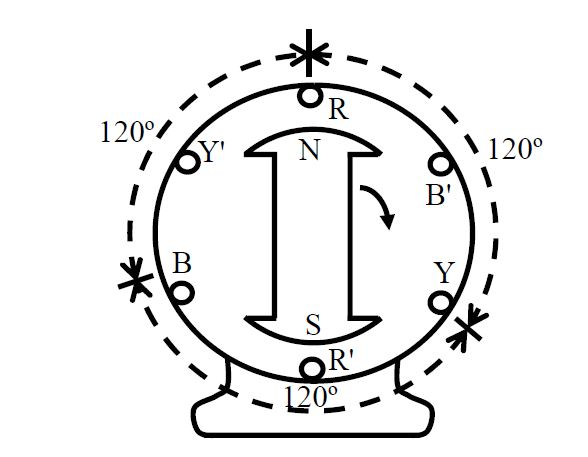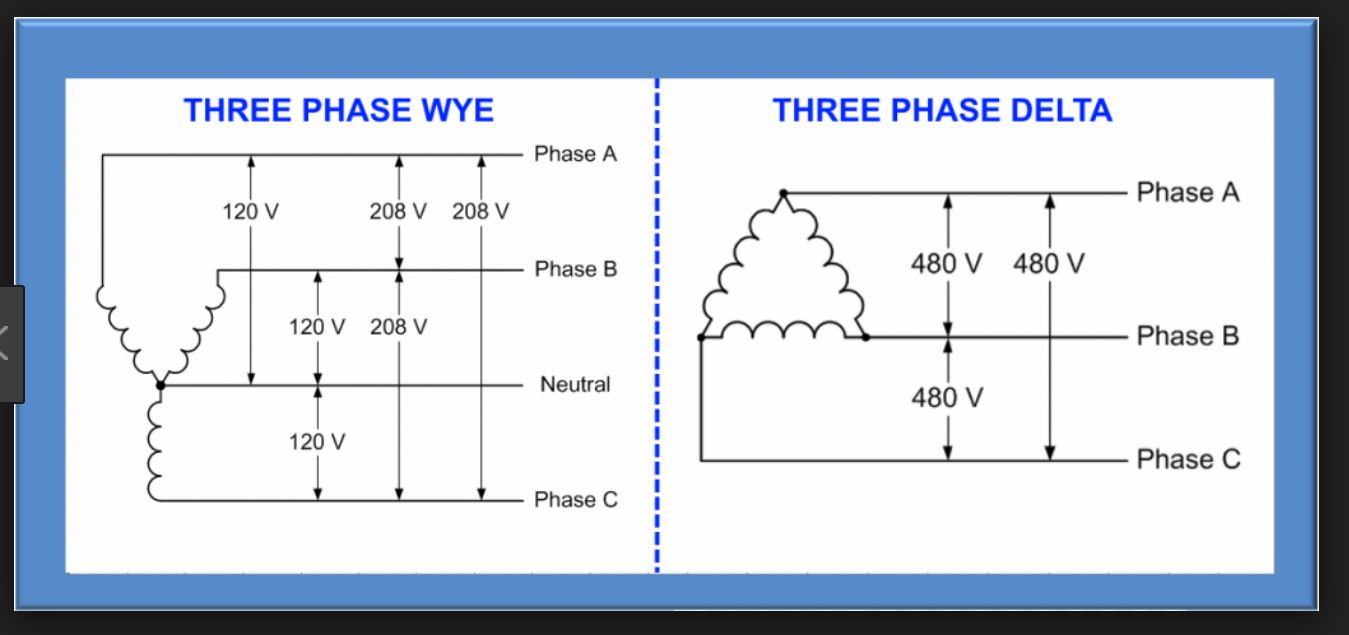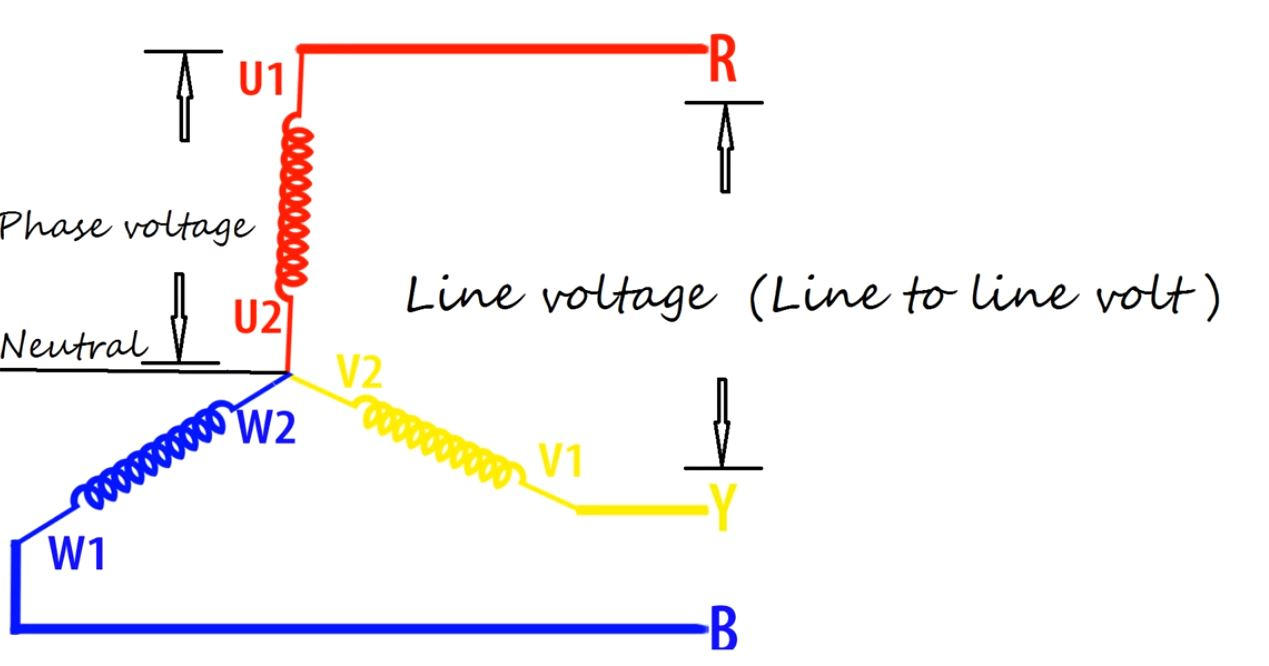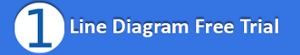During our presentations for the Florida Engineering Society's CEU program this fall, there was one question that was followed by a lively discussion.

The question: What is meant by Line Quantity versus Phase Quantity?

The quantity is for the voltage and current in both the supply and load sides for the 2 connections of a balanced power distribution system. These 2 types of connections are Star and Delta.

This seems like a simple question and distinction, but it does cause confusion. As a basic concept of electrical engineering, it is worth continuing the discussion.

To generate a 3 phase voltage power distribution system, the windings are placed at an electrical angle of 120 degrees from each other. The wave forms in each of the 3 windings (A, B,and C) are shown in Fig. 1.

These windings are in the stator with the poles shown in the rotor. The rotor is rotating at a synchronous speed Ns (rpm (revolutions per minute)). To obtain the frequency (f), the following formula is applied:

f  =  (p x Ns) / 120

where f is frequency and

where p is the number of poles (p = 2)

The 3 phase power distribution system can be connected in either Star or Delta configurations. See Fig. 2The 3 voltages swing up and down similarly to each other, but one after another in a rhythm.

At the receiving end of the electrical load, these 3 lines can be joined together in 2 different ways: (1) a Star (also called a Y connection) or (2) a Delta connection. When the connection is made as a Star, a central point can be established. This point is called neutral, and the sum of all 3 currents will be zero for a perfectly balanced power distribution system.

The neutral point is neutral in the sense that its voltage does not fluctuate like the Phase Voltage. In order to make the neutral point more stable, we can safely connect this point to the ground. Once we have a 3 line terminal and a neutral terminal, we can have 2 different kinds of voltage.A higher voltage between any 2 lines is called Line Voltage or Line-to-Line Voltage. A lower voltage between any 1 line and the neutral is called a Phase Voltage or Line-to-Neutral Voltage. See Figure 3.

Just as there is Line Voltage and Phase Voltage, there is also Line Current and PhaseCurrent. Line Current is the current through any 1 incoming line and Phase Current through any one of the 3 arms of the electrical load.

In the case of the Delta connection, the Line Voltage and Phase Voltage are the same, but the Line Current is √ 3 times the Phase Current.

In the case of the Star Connection, the Line Voltage is √ 3 times the Phase Voltage, but the Line and Phase Currents are the same.

Whether a Star or Delta Connection is used, the same formula (below) is applied to a 3 phase circuit:

P =  √ 3 x line voltage x line current times cos ø (power factor).

where P is Power

In our next article, we will discuss the different electrical service configurations in the United States.